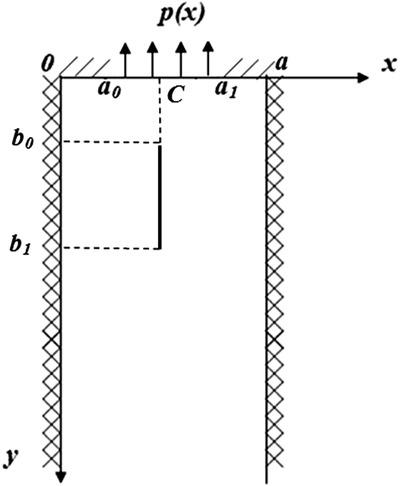ZAMM - Journal of Applied Mathematics and Mechanics ( IF 1.103 ) Pub Date : 2020-01-24 , DOI: 10.1002/zamm.201900289
N. Vaysfeld; Z. ZhuravlovaThe article is dedicated to the investigation of the stress state of a semi‐strip weakened by a longitudinal crack. Two statements of the problem are considered. The integral Fourier transform is applied directly to the initial problem. The discontinuous boundary problem which is formulated in vector form is solved with the help of the matrix differential calculation and the Green's matrix‐function's discontinuous properties. The solving of the problem is reduced to the solving of the system of three singular integral equations (SSIE). This system is solved by two methods regarding to the conditions at the semi‐strip's short edge. The orthogonal polynomials method is used when the semi‐strip is loaded at the center of its short edge, and the special generalized scheme, which allows consideration of fixed singularities, this is applied when the whole semi‐strip's short edge is loaded. The stress intensity factors (SIF) are investigated for the two cases.

down
wechat
bug